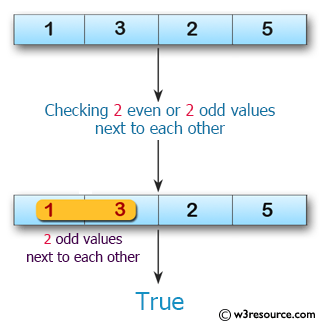﻿ Swift Array Programming Exercise: Test if a given array of integers contains either 2 even or 2 odd values all next to each other - w3resource# Swift Array Programming Exercises: Test if a given array of integers contains either 2 even or 2 odd values all next to each other

## Swift Array Programming: Exercise-34 with Solution

Write a Swift program to test if a given array of integers contains either 2 even or 2 odd values all next to each other.

Pictorial Presentation:Sample Solution:

Swift Code:

``````func two_even_odd(array_nums: [Int]) -> Bool {
for x in 0..<array_nums.count-2 {
if (array_nums[x] % 2 == 0 && array_nums[x+1] % 2 == 0) || (array_nums[x] % 2 == 1 && array_nums[x+1] % 2 == 1) {
return true
}
}
return false
}

print(two_even_odd(array_nums: [2, 1, 3, 5]))
print(two_even_odd(array_nums: [2, 1, 2, 5]))
print(two_even_odd(array_nums: [2, 4, 2, 5]))
print(two_even_odd(array_nums: [1, 3, 2, 5]))
```
```

Sample Output:

```true
false
true
true
```

Swift Programming Code Editor:

Improve this sample solution and post your code through Disqus

What is the difficulty level of this exercise?

﻿